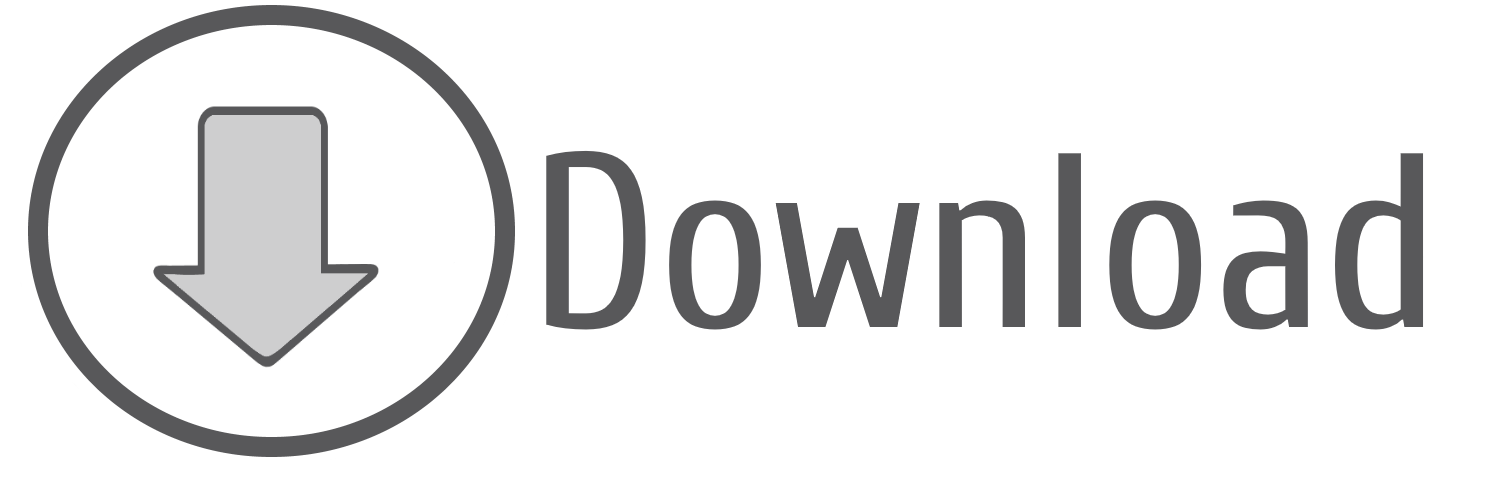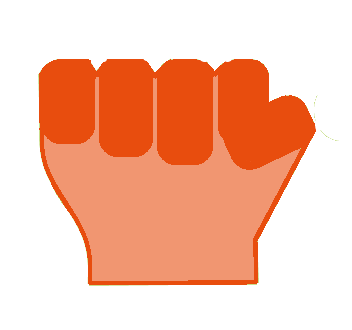Rock, Paper or Scissor Game - Train and Classify [Volume 2]
Difficulty Level:
 Tags train_and_classify☁machine-learning☁features☁extraction

Previous Notebooks that are part of "Rock, Paper or Scissor Game - Train and Classify" module

Following Notebooks that are part of "Rock, Paper or Scissor Game - Train and Classify" module

 ☌ After the presentation of data acquisition conditions on the previous Jupyter Notebook, we will follow our Machine Learning Journey by specifying which features will be extracted. "Features" are numerical parameters extracted from the training data (in our case physiological signals acquired when executing gestures of "Rock, Paper or Scissor" game), characterizing objectively the training example. A good feature is a parameter that has the ability to separate the different classes of our classification system, i.e, a parameter with a characteristic range of values for each available class.

Starting Point (Setup)

List of Available Classes:

1. "No Action" [When the hand is relaxed]
2. "Paper" [All fingers are extended]
3. "Rock" [All fingers are flexed]
4. "Scissor" [Forefinger and middle finger are extended and the remaining ones are flexed]Paper Rock Scissor

Acquired Data:

• Electromyography (EMG) | 2 muscles | Adductor pollicis and Flexor digitorum superficialis
• Accelerometer (ACC) | 1 axis | Sensor parallel to the thumb nail (Axis perpendicular)

Protocol/Feature Extraction

Extracted Features

Formal definition of parameters
☝ | Maximum Sample Value of a set of elements is equal to the last element of the sorted set

☉ | $\mu = \frac{1}{N}\sum_{i=1}^N (sample_i)$

☆ | $\sigma = \sqrt{\frac{1}{N}\sum_{i=1}^N(sample_i - \mu_{signal})^2}$

☌ | $zcr = \frac{1}{N - 1}\sum_{i=1}^{N-1}bin(i)$

☇ | $\sigma_{abs} = \sqrt{\frac{1}{N}\sum_{i=1}^N(|sample_i| - \mu_{signal_{abs}})^2}$

☍ | $m = \frac{\Delta signal}{\Delta t}$

... being $N$ the number of acquired samples (that are part of the signal), $sample_i$ the value of the sample number $i$, $signal_{abs}$ the absolute signal, $\Delta signal$ is the difference between the y coordinate of two points of the regression curve and $\Delta t$ the difference between the x (time) coordinate of the same two points of the regression curve.

... and

$bin(i)$ a binary function defined as:

$bin(i) = \begin{cases} 1, & \mbox{if } signal_i \times signal_{i-1} \leq 0 \\ 0, & \mbox{if } signal_i \times signal_{i-1}>0 \end{cases}$

0 - Import of the needed packages for a correct execution of the current Jupyter Notebook

In :
# Package that ensures a programatically interaction with operating system folder hierarchy.
from os import listdir

# Package used for clone a dictionary.
from copy import deepcopy

# Functions intended to extract some statistical parameters.
from numpy import max, std, average, sum, absolute

# With the following import we will be able to extract the linear regression parameters after
# fitting experimental points to the model.
from scipy.stats import linregress

# biosignalsnotebooks own package that supports some functionalities used on the Jupyter Notebooks.
import biosignalsnotebooks as bsnb


1 - Loading of all signals that integrates our training samples (storing them inside a dictionary)

The acquired signals are stored inside a folder which can be accessed through a relative path "/signal_samples/classification_game/data"

1.1 - Identification of the list of files/examples

In :
# Transposition of data from signal files to a Python dictionary.
relative_path = "/signal_samples/classification_game"
data_folder = "data"

# List of files (each file is a training example).
list_examples = listdir(relative_path + "/" + data_folder)

In :
print(list_examples)

['0_1.txt', '0_2.txt', '0_3.txt', '0_4.txt', '0_5.txt', '1_1.txt', '1_2.txt', '1_3.txt', '1_4.txt', '1_5.txt', '2_1.txt', '2_2.txt', '2_3.txt', '2_4.txt', '2_5.txt', '3_1.txt', '3_2.txt', '3_3.txt', '3_4.txt', '3_5.txt']


The first digit of filename identifies the class to which the training example belongs and the second digit is the trial number ( <class>_<trial>.txt )

1.2 - Access the content of each file and store it on the respective dictionary entry

In :
# Initialization of dictionary.
signal_dict = {}

# Scrolling through each entry in the list.
for example in list_examples:
if ".txt" in example: # Read only .txt files.
# Get the class to which the training example under analysis belong.
example_class = example.split("_")

# Get the trial number of the training example under analysis.
example_trial = example.split("_").split(".")

# Creation of a new "class" entry if it does not exist.
if example_class not in signal_dict.keys():
signal_dict[example_class] = {}

complete_data = bsnb.load(relative_path + "/" + data_folder + "/" + example)

# Store data in the dictionary.
signal_dict[example_class][example_trial] = complete_data


1.3 - Definition of the content of each channel

In :
# Channels (CH1 Flexor digitorum superficialis | CH2 Aductor policis | CH3 Accelerometer axis Z).
emg_flexor = "CH1"
acc_z = "CH3"


2 - Extraction of features according to the signal under analysis

The extracted values of each feature will be stored in a dictionary with the same hierarchical structure as "signal_dict"

In :
# Clone "signal_dict".
features_dict = deepcopy(signal_dict)

# Navigate through "signal_dict" hierarchy.
list_classes = signal_dict.keys()
for class_i in list_classes:
list_trials = signal_dict[class_i].keys()
for trial in list_trials:
# Initialise "features_dict" entry content.
features_dict[class_i][trial] = []

for chn in [emg_flexor, emg_adductor, acc_z]:
# Temporary storage of signal inside a reusable variable.
signal = signal_dict[class_i][trial][chn]

# Start the feature extraction procedure accordingly to the channel under analysis.
if chn == emg_flexor or chn == emg_adductor: # EMG Features.
# Converted signal (taking into consideration that our device is a "biosignalsplux", the resolution is
# equal to 16 bits and the output unit should be in "mV").
signal = bsnb.raw_to_phy("EMG", device="biosignalsplux", raw_signal=signal, resolution=16, option="mV")

# Standard Deviation.
features_dict[class_i][trial] += [std(signal)]
# Maximum Value.
features_dict[class_i][trial] += [max(signal)]
# Zero-Crossing Rate.
features_dict[class_i][trial] += [sum([1 for i in range(1, len(signal))
if signal[i]*signal[i-1] <= 0]) / (len(signal) - 1)]
# Standard Deviation of the absolute signal.
features_dict[class_i][trial] += [std(absolute(signal))]
else: # ACC Features.
# Converted signal (taking into consideration that our device is a "biosignalsplux", the resolution is
# equal to 16 bits and the output unit should be in "g").
signal = bsnb.raw_to_phy("ACC", device="biosignalsplux", raw_signal=signal, resolution=16, option="g")

# Average value.
features_dict[class_i][trial] += [average(signal)]
# Standard Deviation.
features_dict[class_i][trial] += [std(signal)]
# Maximum Value.
features_dict[class_i][trial] += [max(signal)]
# Zero-Crossing Rate.
features_dict[class_i][trial] += [sum([1 for i in range(1, len(signal))
if signal[i]*signal[i-1] <= 0]) / (len(signal) - 1)]
# Slope of the regression curve.
x_axis = range(0, len(signal))
features_dict[class_i][trial] += [linregress(x_axis, signal)]


Each training array has the following structure/content:
[$\sigma_{emg\,flexor}$, $max_{emg\,flexor}$, $zcr_{emg\,flexor}$, $\sigma_{emg\,flexor}^{abs}$, $\sigma_{emg\,adductor}$, $max_{emg\,adductor}$, $zcr_{emg\,adductor}$, $\sigma_{emg\,adductor}^{abs}$, $\mu_{acc\,z}$, $\sigma_{acc\,z}$, $max_{acc\,z}$, $zcr_{acc\,z}$, $m_{acc\,z}$]

3 - Storage of the content inside the filled "features_dict" to an external file ( .json)

With this procedure it is possible to ensure a "permanent" memory of the results produced during feature extraction, reusable in the future by simple reading the file (without the need to reprocess again).

In :
# Package dedicated to the manipulation of json files.
from json import dump

filename = "classification_game_features.json"

# Generation of .json file in our previously mentioned "relative_path".
# [Generation of new file]
with open(relative_path + "/features/" + filename, 'w') as file:
dump(features_dict, file)


We reach the end of the "Classification Game" second volume. Now all the features of training examples are in our possession. If you are feeling your interest increasing, please jump to the next volumeWe hope that you have enjoyed this guide. biosignalsnotebooks is an environment in continuous expansion, so don"t stop your journey and learn more with the remaining Notebooks!☌ Project Presentation ☌ GitHub Repository ☌ How to install biosignalsnotebooks Python package ? ☌ Signal Library ☌ Notebook Categories ☌ Notebooks by Difficulty ☌ Notebooks by Signal Type ☌ Notebooks by Tag
In :
from biosignalsnotebooks.__notebook_support__ import css_style_apply
css_style_apply()

.................... CSS Style Applied to Jupyter Notebook .........................

Out: